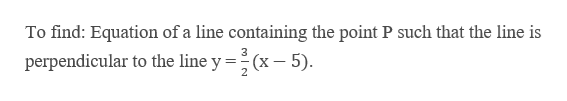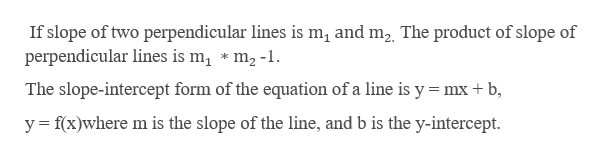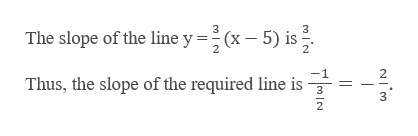# Find the equation of the line containing the pointP(2, 3)and perpendicular to the graph ofy = 3/2x − 5.

Question
98 views

Find the equation of the line containing the point

P(2, 3)

and perpendicular to the graph of

y = 3/2x − 5.

check_circle

Step 1

Given a point P (2, 3)help_outlineImage TranscriptioncloseTo find: Equation of a line containing the point P such that the line is 2 perpendicular to the line y(x -5) fullscreen
Step 2

Note:help_outlineImage TranscriptioncloseIf slope of two perpendicular lines is m2 and m2 The product of slope of perpendicular lines is mi * m2-1 The slope-intercept form of the equation of a line is y mx b yf(x)where m is the slope of the line, and b is the y-intercept fullscreen
Step 3

Calculate:&n...help_outlineImage Transcriptionclose3 The slope of the line (x-5) is Thus, the slope of the required line is 2 fullscreen

### Want to see the full answer?

See Solution

#### Want to see this answer and more?

Solutions are written by subject experts who are available 24/7. Questions are typically answered within 1 hour.*

See Solution
*Response times may vary by subject and question.
Tagged in

### Other